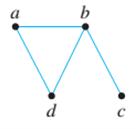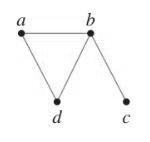Chapter 10.6, Problem 1ES### Discrete Mathematics With Applicat...

5th Edition
EPP + 1 other
ISBN: 9781337694193

#### Solutions

Chapter
Section### Discrete Mathematics With Applicat...

5th Edition
EPP + 1 other
ISBN: 9781337694193
Textbook Problem
24 views

# Find all possible spanning trees for each of the graphs in 1 and 2.1.To determine

To find:

All the possible spanning trees for the given graph.Explanation

Given information:

The given graph is shown below:

Definition Used:

Spanning Tree:

A spanning tree for a graph G is a sub-graph of G that contains every vertex of G and is a tree. A spanning tree does not have cycles and it cannot be disconnected graph.

The given graph G has one circuit dabd and removal of any edges of the circuit gives a tree.

The complete graph can have maximum nn2 number of spanning trees.

The complete graph dabd is as shown below:

### Still sussing out bartleby?

Check out a sample textbook solution.

See a sample solution

#### The Solution to Your Study Problems

Bartleby provides explanations to thousands of textbook problems written by our experts, many with advanced degrees!

Get Started